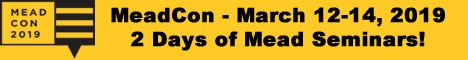1.Calculation Formulae (SG, %ABV,...)

From somewhere else in the Forum

SPECIFIC GRAVITY from HONEY quantity

SG = 1 + [ (lbs x 45) / (gals x 1000) ]

(and for metric people)

SG = 1 + [ (Kg) / (Ltr x 2.6596) ]

POTENTIAL ALCOHOL from SG

(This allows for FG < 1.000 - see Ben Rotter's site http://members.tripod.com/~BRotter/C...o/HydSugAl.htm )

%ABV = 0.944 + 129.8 x (SG - 1)

Any other handy formulae out there?Reply With Quote

2.Piping QueenJoin Date
Aug 2004
Posts
600Re: Calculation Formulae (SG, %ABV,...)

POTENTIAL ALCOHOL from SG

(This allows for FG < 1.000 - see Ben Rotter's site http://members.tripod.com/~BRotter/C...o/HydSugAl.htm )

%ABV = 0.944 + 129.8 x (SG - 1)

Any other handy formulae out there?
Would I be correct in assuming that should be
%ABV = 0.944 + [129.8 x (SG-1)] ?Reply With Quote

3.Worker BeeJoin Date
Nov 2003
Posts
378Re: Calculation Formulae (SG, %ABV,...)

My rule of thumb is 1 lb of honey in 5 gals of water = 0.008 SG = 1% ABV fermented out. Simple, pretty close to reality, and much easier to remember than those equations.I have a question about that SG to ABV formula. If I plug in a SG of 1 (= water), I get a ABV% of .994%. What gives?Reply With Quote

4.Piping QueenJoin Date
Aug 2004
Posts
600Re: Calculation Formulae (SG, %ABV,...)

My rule of thumb is 1 lb of honey in 5 gals of water = 0.008 SG = 1% ABV fermented out. Simple, pretty close to reality, and much easier to remember than those equations.I have a question about that SG to ABV formula. If I plug in a SG of 1 (= water), I get a ABV% of .994%. What gives?
I guess that's what happens when you use a simplified equation to get an approximation. On the "Mead Made complicated" site they give the actual equations to figure this out, those equations frighten and confuse meReply With Quote

5.Re: Calculation Formulae (SG, %ABV,...)

WikdWaze,

The brackets were left out because I assumed muiltiplication has precedence over additionGoes to show what assuming does.

Scott S,

Plugging in SG=1.000 says that your FG=1.000, and your mead isn't totally dry, but still has 0.944% Alc to ferment out.
When your FG=0.9927 you will have a totally dry mead. Again this is another assumption, because EC-1118 can probably ferment to FG=0.9927, but some of the other yeasts may not. However, we are talking about potential alcoholI'm sure you didn't start with OG=1.000The URL I mentioned has more details, and there are 5 formulae listed there, each with slightly different results.

-=-=-=-

1lb in 5 gal making 1% ABV - that is an easy one to remember, except for the "metric" people :-/

that makes it (roughly) 0.4 Kg in 19 Litres per 1% ABVReply With Quote

6.Re: Calculation Formulae (SG, %ABV,...)

Nice references James!Good information!

OskaarReply With Quote

7. jabPiping QueenJoin Date
Mar 2004
Posts
557Re: Calculation Formulae (SG, %ABV,...)

I started writing a PHP application a while back that would allow me to track my brews. Life got in the way and I didn't get very far on it, however, this is the function I was using to figure alcohol content either by weight or vol.

Code:
```<?

function figureAlcohol(\$ograv, \$fgrav, \$pmode = 'vol')
{
\$alc = ((\$ograv - \$fgrav) * 105);

if (\$pmode == 'vol')
{
\$alc *= 1.25;
}

return \$alc;
}

?>```
Hope that helps someone.

-jbReply With QuoteRe: Calculation Formulae (SG, %ABV,...)

I developed a little spreadsheet that helps you formulate simple recipes, if anybody's interested. Just PM me with your e-mail and I'll send it to you.

BReply With QuotePosting Permissions

• You may not post new threads
• You may not post replies
• You may not post attachments
• You may not edit your posts
•